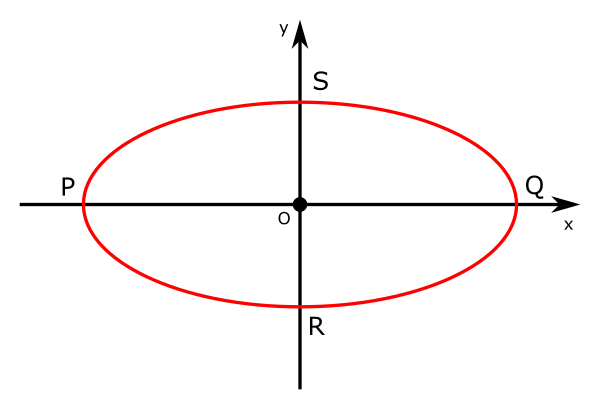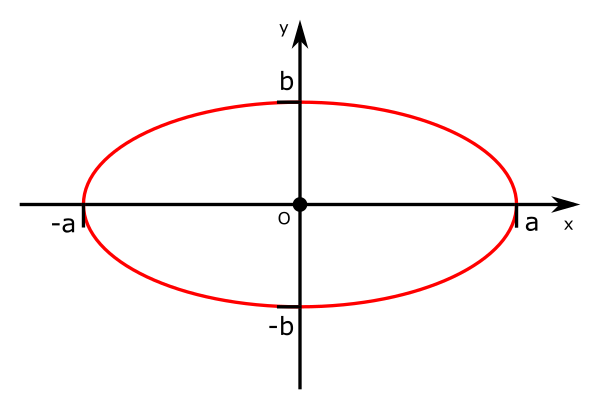# Ellipses

Martin McBride, 2020-09-14
Tags ellipse major axis minor axis
Categories coordinate systems pure mathematics

An ellipse is a stretched circle.The longest diameter, $PQ$, is called the major axis. The shortest diameter, $RS$, is called the minor axis. The major and minor axes are always perpendicular.

An ellipse can be defined by the parameters $a$ and $b$, where the major axis has length $2a$ and the minor axis has length $2b$ (or vice versa). An ellipse centred on the origin with its major and minor axes aligned with the x and y axes looks like this:The ellipse crosses the x axis at points (a, 0) and (-a, 0). It crosses the y axis at points (0, b) and (0, -b).

A circle is a special case of an ellipse, where the major and minor axes have the same length.